# Ray Tracing in Monochromators and Spectrometers

December 25, 2014

Optical devices such as monochromators and spectrometers can be used to separate polychromatic, or multicolored, light into separate colors. These devices have many applications in diverse areas that range from chemistry to astronomy. Using built-in tools in the Ray Optics Module, it is possible to model the separation of electromagnetic rays at different frequencies with a monochromator or spectrometer as well as analyze the resolution of such devices.

### The Setup of a Basic Spectrometer

A spectrometer is a device that measures some property of radiation (e.g., its intensity or state of polarization) as a function of its frequency. Spectrometers can be designed for detecting radiation at a number of different frequency ranges. This extends from visible light to gamma rays and infrared radiation.

A basic spectrometer includes a lens or mirror to convert incoming light into a parallel (or collimated) beam along with a mechanism for separating the light into different frequencies. The device also features another lens or mirror to focus light of different frequencies at specific locations. If a narrow exit slit is used to only transmit radiation of a specific frequency, the device is referred to as a monochromator.

Spectrometers are widely used to analyze the composition of mixtures of chemicals. Each element releases photons in specific frequency ranges (collectively known as the element’s emission spectrum) as excited electrons return to lower energy states. Using known emission spectra, it is possible to determine the composition of a sample based on the radiation that it emits. Similarly, it is possible to analyze the composition of stars based on the radiation they emit and even estimate the redshift of very distant objects.

There are several ways to separate polychromatic light into individual colors. Early spectrometers often used a prism made of a material with a frequency-dependent refractive index n. Such materials are also called dispersive media. As light enters and leaves the prism, its direction of propagation is determined by Snell’s Law,

(1)

n_i \sin(\theta_i) = n_t \sin(\theta_t)

where the subscripts i and t denote the incident and refracted light, respectively. If the refractive index is frequency-dependent and the angle of incidence is nonzero, then the angle of refraction is also frequency-dependent. As a result, a collimated beam of polychromatic light will be separated, as illustrated below.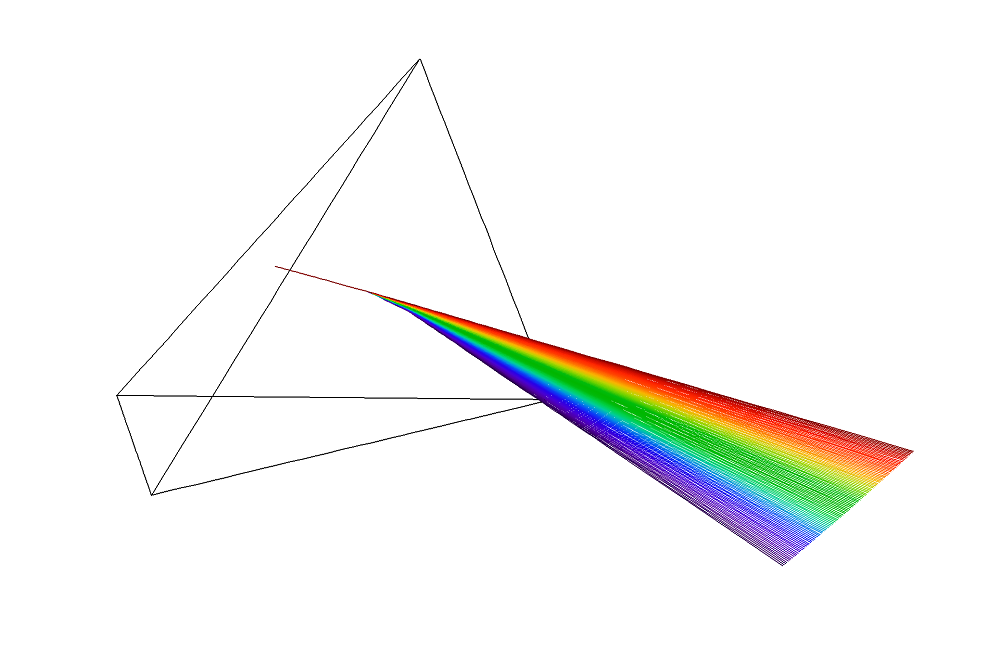Modern spectrometers typically use a diffraction grating instead of a prism containing a dispersive medium. A diffraction grating is a periodic arrangement containing a large number of identical unit cells. When an electromagnetic wave reaches the grating, it can only be transmitted and reflected in specific directions. These directions depend on the wavelength of the incident light and the width of a single unit cell d.

In order for reflected or refracted light to propagate in a certain direction, the waves from adjacent unit cells must interfere constructively with each other. For a reflected ray of free-space wavelength \lambda_0 with integer-valued diffraction order m, the angle of incidence \theta_i and angle of reflection \theta_r are related by

(2)

m\lambda_0 = d n_1 \left(\sin\theta_r -\sin\theta_i\right)

The reflection of rays from adjacent unit cells is illustrated below.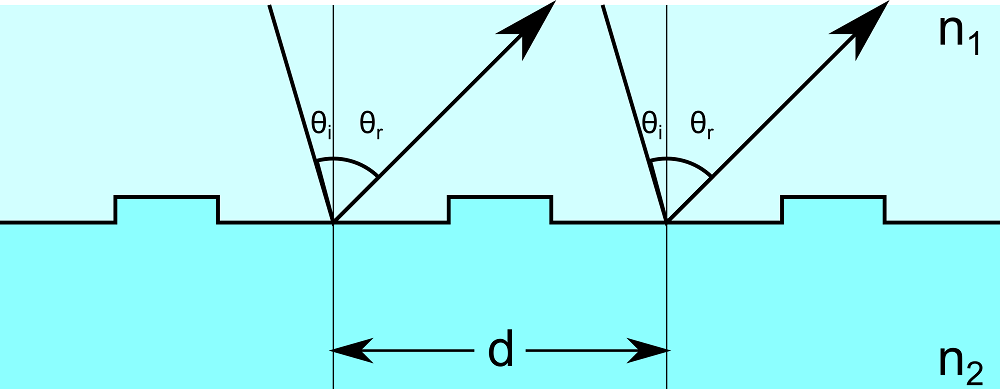From Eq. (2), it is clear that if the diffraction order is nonzero, the direction of reflected radiation will depend on the free-space wavelength. This is the basic property of the grating that can be used to separate different frequencies of radiation.

### Modeling the Czerny-Turner Configuration

We now use the COMSOL Multiphysics® software with the Ray Optics Module to model light propagation in a basic optical device. This example consists of two mirrors and a diffraction grating arranged in a crossed Czerny-Turner configuration, which is depicted below.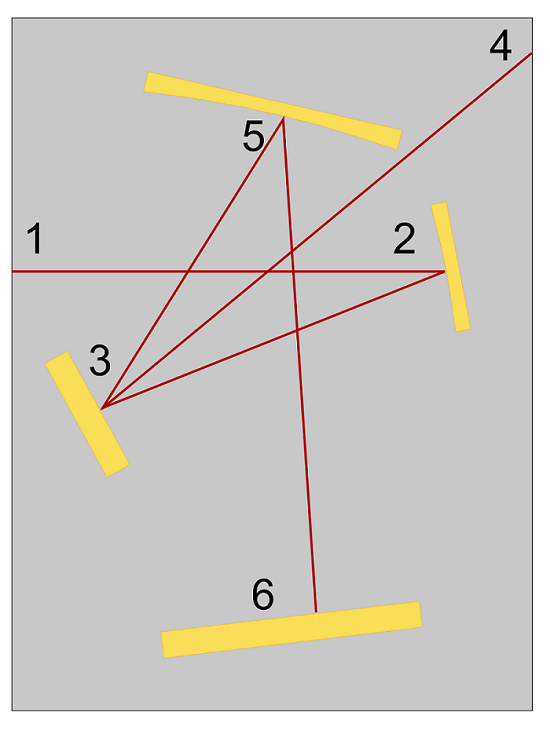Incoming rays are released from a slit (1) with a conical distribution. The rays are reflected by a collimating mirror (2) so that all of the rays are parallel as they hit the diffraction grating (3). The reflected rays of diffraction order 0 travel along parallel paths because their angle of reflection is not wavelength-dependent. Because the different colors have not been separated, these rays are aimed away from the mirrors and ignored (4).

However, the rays of diffraction order 1 are reflected in different directions based on free-space wavelength. They are reflected by a focusing mirror (5) so that rays of different frequencies are focused at different points on a detector (6). If a narrow exit slit were placed at the detector, the resulting device would be a Czerny-Turner monochromator capable of transmitting radiation only in an extremely narrow frequency band.

In order to compute the ray paths as accurately as possible, we can resolve the mesh on the curved surfaces of the collimating mirror and the focusing mirror. On planar surfaces, a coarse mesh is acceptable. One way to quickly set up the mesh is to specify a very low curvature factor, which causes the mesh to be automatically refined near curved boundaries.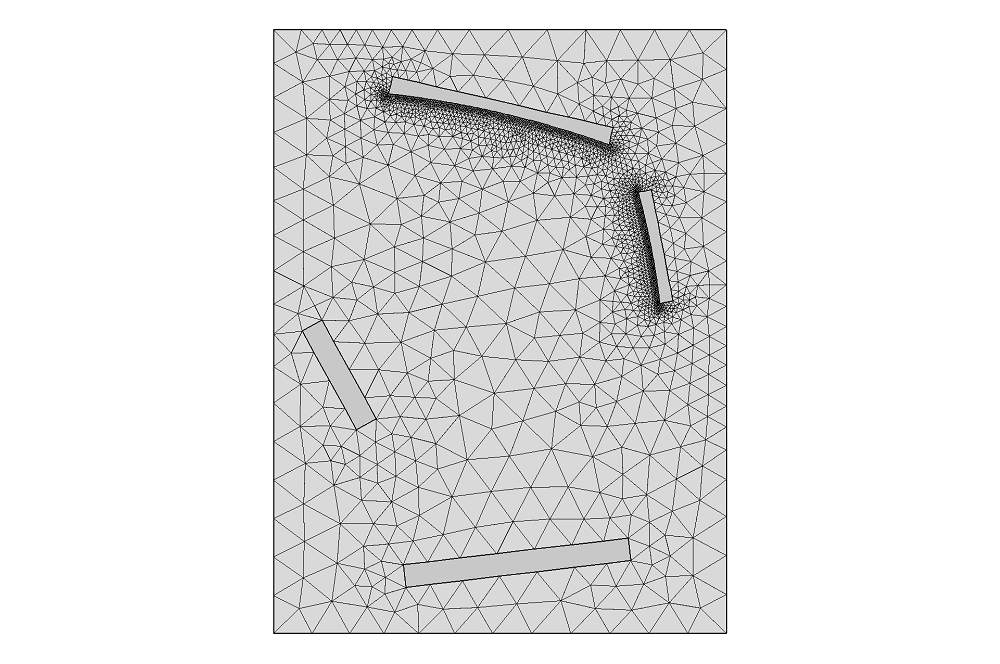The paths followed by rays of different colors are shown in the following plot.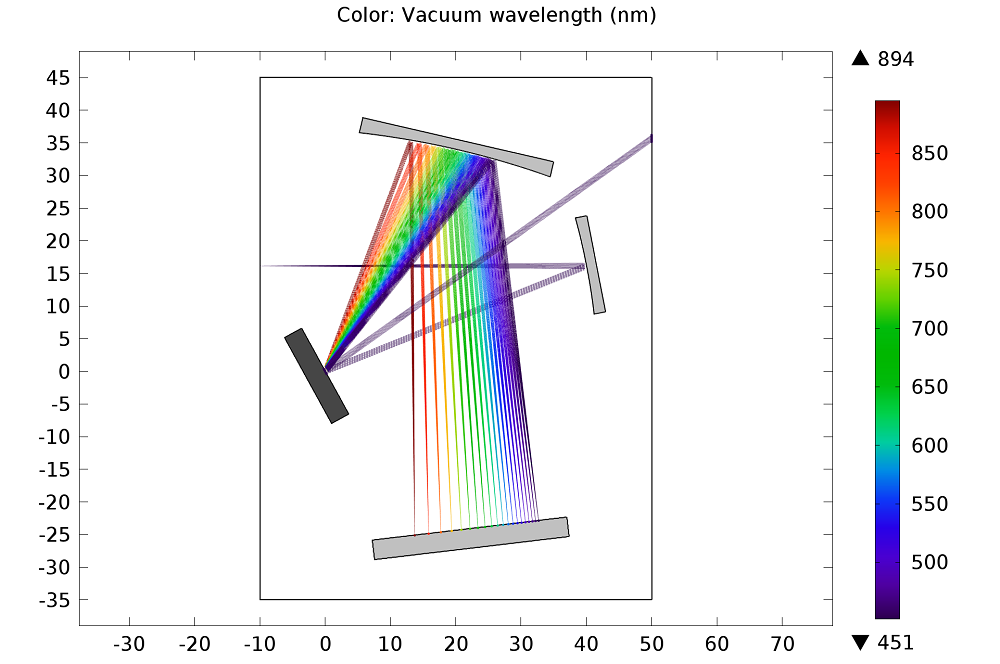Although the crossed Czerny-Turner configuration appears to focus rays of each frequency to a distinct point, rays of a single frequency are actually distributed over an area of small but nonzero width. We can see this more clearly by zooming in at the detector surface.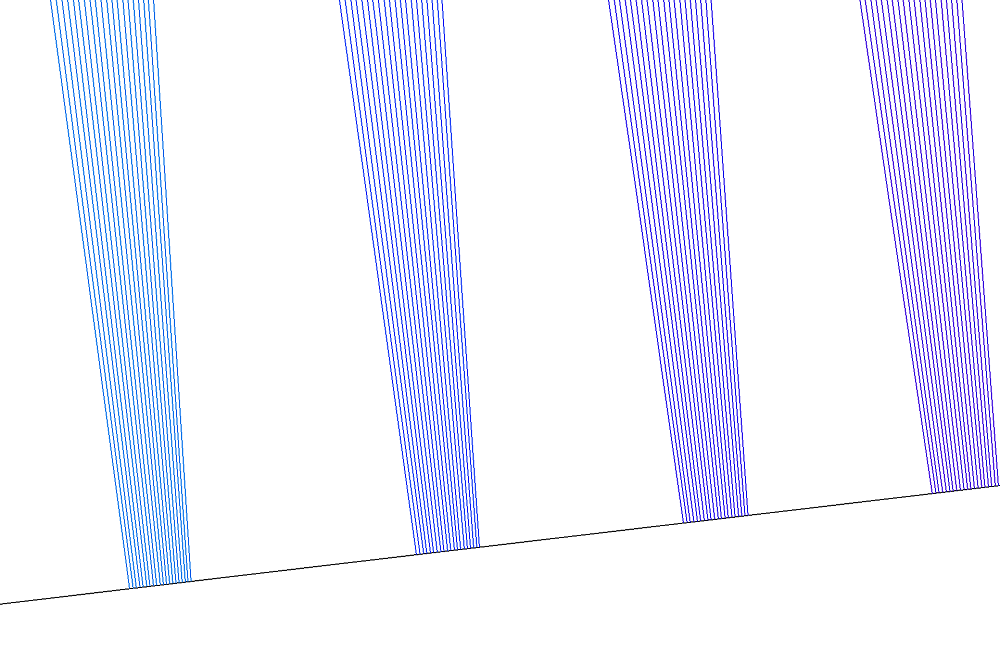It is clear that rays of a single frequency are not focused to a single point. This naturally leads us to wonder about the resolution of the device. In other words, using the mirrors and grating as they are arranged in this model, what is the smallest change in wavelength that we can detect? It is possible to analyze the resolution using the Ray plot type. One way to quantify the resolution of the device is by using the expression

(3)

\textrm{Resolution} = \frac{Sw_{i}}{Nw_{p}}

where S is the spectral width of the incident polychromatic beam, w_i is the width of an incident monochromatic beam at the detector, w_p is the width of a single pixel on the detector, and N is the total number of pixels. The resulting resolution is shown in the following plot.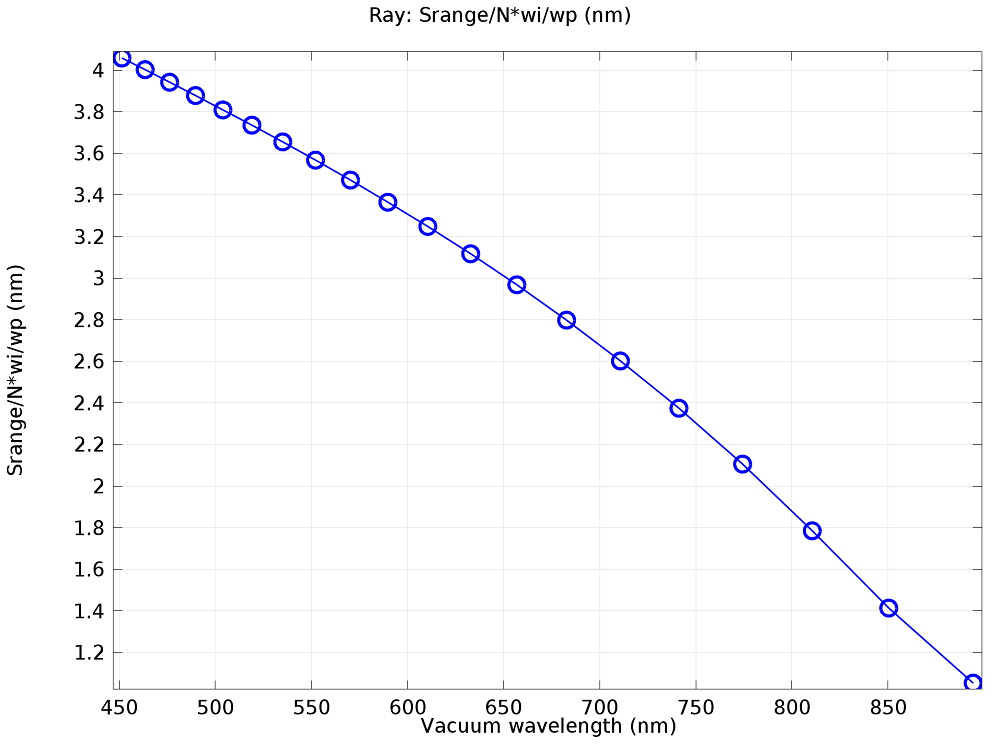### Next Steps

#### Comentários (1)

##### Faça um Comentário
Carregando...##### Naif Alsalem
November 22, 2019

Hi Chris,
Thank you for this nicely illustrated tutorial of CT spectrometer.
I would like to ask how did you determine the spectral range in the model? because what I see in the parameters table is one parameter named: Srange and its value is 650nm.
If I want to define my spectral range to be, for example, from 1700nm to 2300nm, how could I do this?

Thank you very much in advance
Best
Naif

EXPLORE O BLOG COMSOL